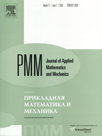Journal of AppliedMathematics and MechanicsRussian Academy of Sciences Founded in January 1936 (Translated from 1958) Issued 6 times a year ISSN 0021-8928(print version)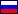Russian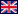English About Journal | Issues | Editorial Board | Contact Us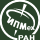Web hosting is providedby the Ishlinsky Institute forProblems in Mechanicsof the RussianAcademy of Sciences
IssuesArchive of Issues2012-6pp.646-657

# Archive of Issues

 Total articles in the database: 2048 In Russian (ПММ): 1249 In English (J. Appl. Math. Mech.): 799
 << Previous article | Volume 76, Issue 6 / 2012 | Next article >> Yu.N. Chelnokov, "Optimal reorientation of a spacecraft's orbit using a jet thrust orthogonal to the orbital plane," J. Appl. Math. Mech. 76 (6), 646-657 (2012) Year 2012 Volume 76 Issue 6 Pages 646-657 Title Optimal reorientation of a spacecraft's orbit using a jet thrust orthogonal to the orbital plane Author(s) Yu.N. Chelnokov (Saratov, Russia, chelnokovyun@gmail.com) Abstract The problem of the optimal control of the orientation of a spacecraft's orbit using magnitude-constrained control, i.e., the jet thrust vector perpendicular to the orbital plane, is considered. Under such control the spacecraft's orbit rotates in space as an unalterable (non-deformable) figure. A combined functional, equal to the weighted sum of the reorientation time and an integral quadratic (with respect to the control) quality functional, or equal to the weighted sum of the reorientation time and the control momentum (the characteristic velocity) during reorientation of the orbit serves as an optimality criterion. A quaternion differential equation of the orientation of the orbital system of coordinates and the maximum principle are used to solve the problem. A differential boundary-value problem with a dimension of ten is formulated to construct the optimal control and the optimal trajectory for reorientating the orbit. Control laws that satisfy the necessary optimality conditions are obtained (in the form of functions of conjugate variables). Quaternion and scalar first integrals of the equations of the problem are found, and transversality conditions that do not contain Lagrange undetermined multipliers are devised. It is shown that the original boundary-value problem reduces (with simultaneous simplification of the equations of the problem) to a new boundary-value problem of a smaller dimension equal the three, whose equations for a circular orbit in the case of maximum speed are integrated in trigonometric functions. Such a reduction is possible owing to the self-conjugation of the quaternion differential equation of the orientation of the orbital system of coordinates of the spacecraft and the use of a new quaternion variable that is related to the quaternion first integral by a rotational transformation. It is found that the switching function of the control is described by a system of three first-order differential equations, which reduce to a linear inhomogeneous second-order equation with constant coefficients for a circular orbit in the time-optimal case and to the Duffing equation in the case of an integral quadratic quality functional. It is also shown that for a circular orbit in the time-optimal case the problem reduces to solving a third-order non-linear algebraic system. An example of a numerical solution of the problem is presented. Received 26 November 2010 Link to Fulltext http://www.sciencedirect.com/science/article/pii/S0021892813000245 << Previous article | Volume 76, Issue 6 / 2012 | Next article >>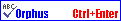If you find a misprint on a webpage, please help us correct it promptly - just highlight and press Ctrl+Enter

 101 Vernadsky Avenue, Bldg 1, Room 245, 119526 Moscow, Russia • (+7 495) 434-2149 • pmm@ipmnet.ru • pmmedit@ipmnet.ru • https://pmm.ipmnet.ru Founders: Russian Academy of Sciences, Branch of Power Industry, Machine Building, Mechanics and Control Processes of RAS, Ishlinsky Institute for Problems in Mechanics RAS © Journal of Applied Mathematics and Mechanics webmaster